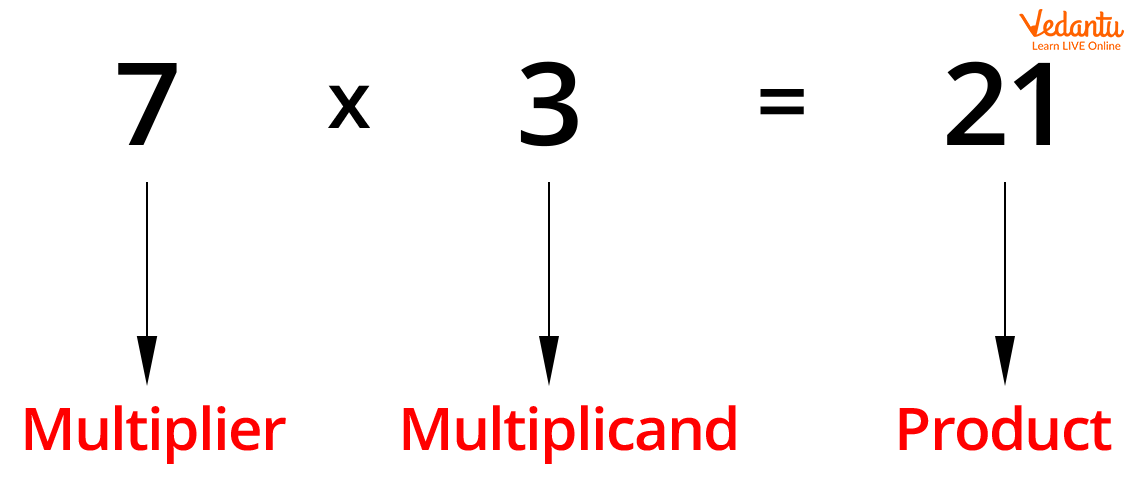Courses
Courses for Kids
Free study material
Free LIVE classes
More

# Multiplier and Multiplicand and Properties of MultiplicationLIVE
Join Vedantu’s FREE Mastercalss

## An Introduction to Multiplier and Multiplicand

There are four basic operations of Mathematics – these are addition, subtraction, multiplication, and division. So, multiplication is one of the elementary operations of computation in Mathematics. In the process of multiplication, the number to be multiplied is termed the multiplicand and the number with which we multiply is called the multiplier. Product is the result of multiplication. In the below given example, the multiplication of 8 and 5 is performed where 8 is the multiplier, 5 is multiplicand, and 40 is the product. There is a ‘’ sign that is used to represent multiplication.Image: Example of multiplication

## Properties of Multiplication

There are some properties of multiplication which are as follows:

1. Commutative Property of Multiplication

According to the commutative property of multiplication, when the order of the multiplier and multiplicand are changed, the product does not change.

For example: 4 × 3 = 12 or 3 × 4 = 12

So, 4 × 3 = 3 × 4.

1. Associative Property of Multiplication

According to the associative property of multiplication, the product of three or more numbers does not change if we change the grouping of the numbers.

For example: (4 × 7) × 5 = 28 × 5 = 140

or, (7 × 5) × 4 = 35 × 4= 140

or, (4 × 5) × 7 = 20 × 7 = 140

1. One Property of Multiplication

According to one property of the multiplication, the product of a number and 1 is the number itself.

For example:

18 × 1 = 18

75 × 1 = 75

54 × 1 = 54

21 × 1 = 21

1. Zero Property of Multiplication

According to the zero property of multiplication, the result of multiplication of any number and zero is zero.

For example:

5 × 0 = 0

0 × 15 = 0

24 × 0 = 0

90 × 0 = 0

1. Distributive Property of Multiplication

According to the distributive property of multiplication, the product of a number and the sum of two numbers is always the same as the sum of the product of the numbers.

For example:

4 × (3 + 5) = 4 × 8 = 32

4 × 3 + 4 × 5 = 32

So, 4 × (3 + 5) = 4 × 3 + 4 × 5 = 32

In the same way, the product of a number and the difference of two numbers is also always the same as the difference of the product of the numbers.

For example:

4 × (6 − 5) = 4

4 × 6 − 4 × 5 = 24 − 20 = 4

So, 4 × (6 − 5) = 4 × 6 − 4 × 5 = 4

## Conclusion

It is important for the students to clearly understand the concept of multiplier and multiplicand. The properties of multiplication are based on multiplier and multiplicand. Every property of multiplication is explained with examples in the above article.

Last updated date: 22nd Sep 2023
Total views: 136.2k
Views today: 1.36k

## FAQs on Multiplier and Multiplicand and Properties of Multiplication

1. What is multiplication?

Multiplication is one of the four elementary Mathematical operations of arithmetic calculations. We know that there are four basic operations in Mathematics which are addition, subtraction, multiplication, and division. The result of the operation of multiplication is termed as product. The multiplication of whole numbers can be understood by considering repeated addition because the multiplication of two numbers is equivalent to adding as many copies of one of them.

2. Which is the multiplier and multiplicand?

While performing Mathematical multiplication operation, two numbers are separated through sign of multiplication that is ‘' or ‘*’ or dot. These two numbers give the product or the result of multiplication. The digit or digits to be multiplied is termed the multiplicand and the digit or digits with which we multiply is called the multiplier.